# Selina Solutions Concise Mathematics Class 6 Chapter 25 Properties of Angles and Lines Exercise 25(C)

Selina Solutions Concise Mathematics Class 6 Chapter 25 Properties of Angles and Lines Exercise 25(C) provides students various methods of solving problems effortlessly. In this exercise, students can follow the important steps to be followed in the construction of angles. The main intention of creating the solutions in a stepwise manner, is to reduce the fear of examination among students. Those who practice these solutions frequently, obtain in-depth knowledge about the concept and also improve academic performance. Here, students can find Selina Solutions Concise Mathematics Class 6 Chapter 25 Properties of Angles and Lines Exercise 25(C) free PDF, from the links which are available below.

## Selina Solutions Concise Mathematics Class 6 Chapter 25: Properties of Angles and Lines Exercise 25(C) Download PDF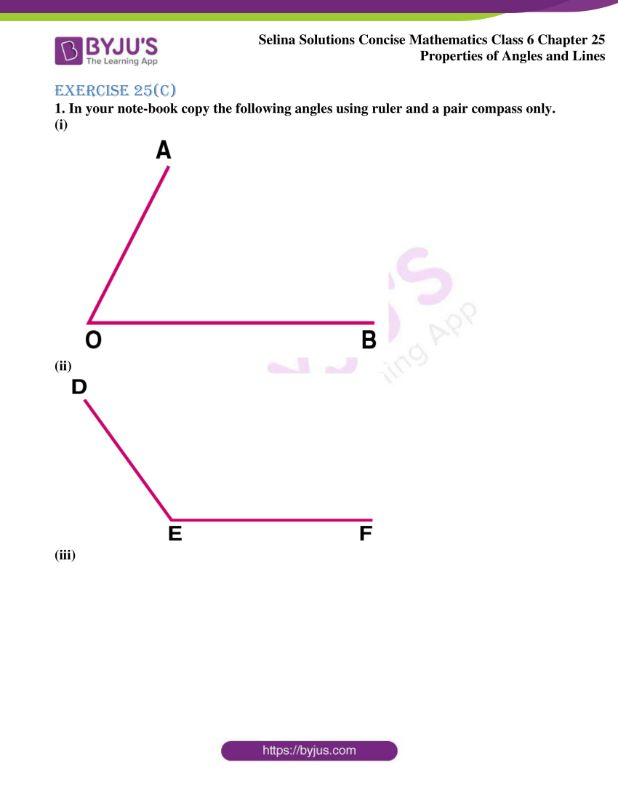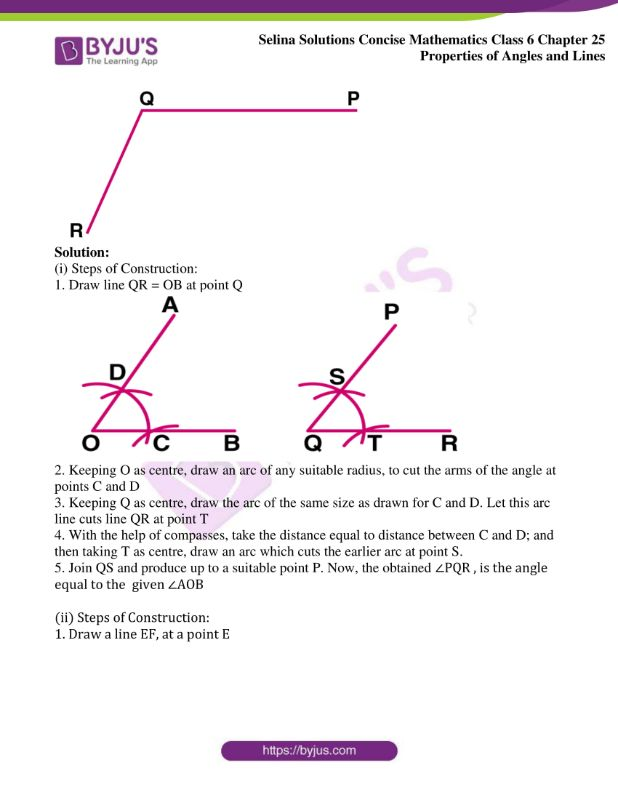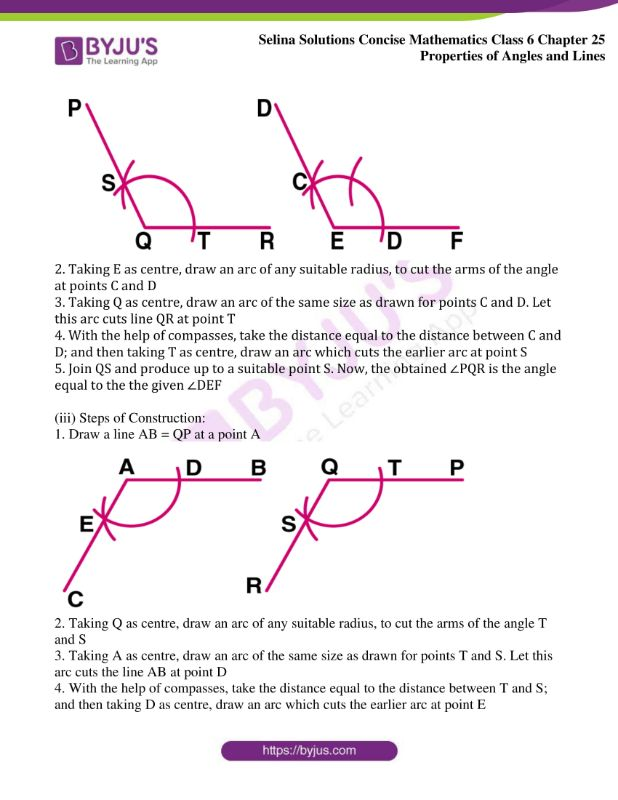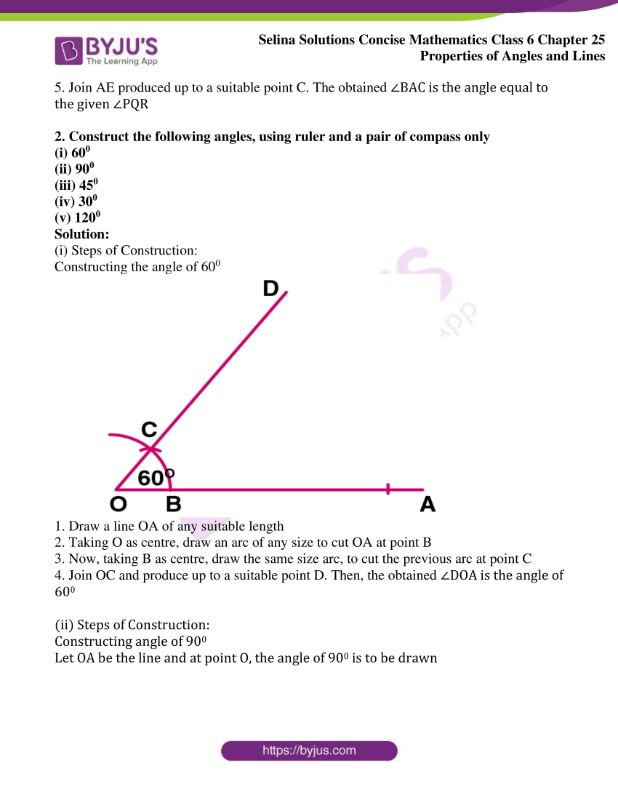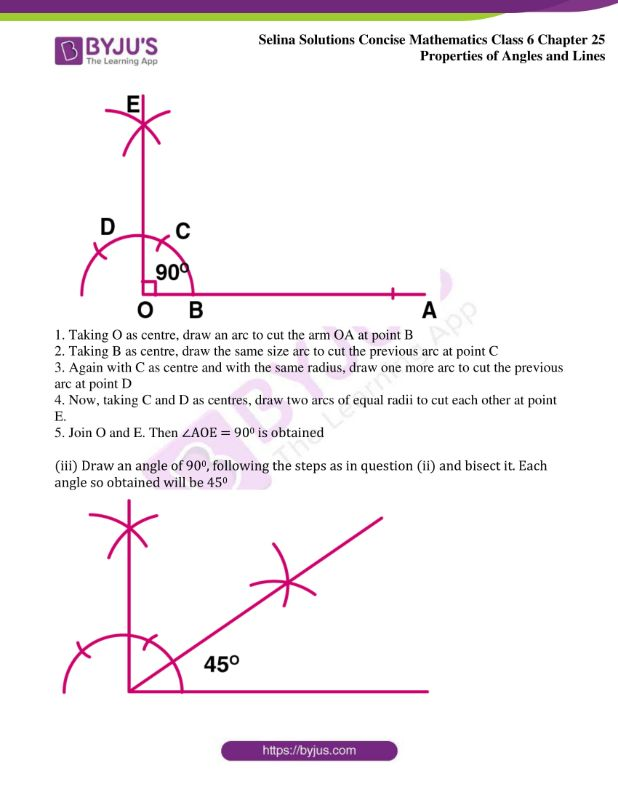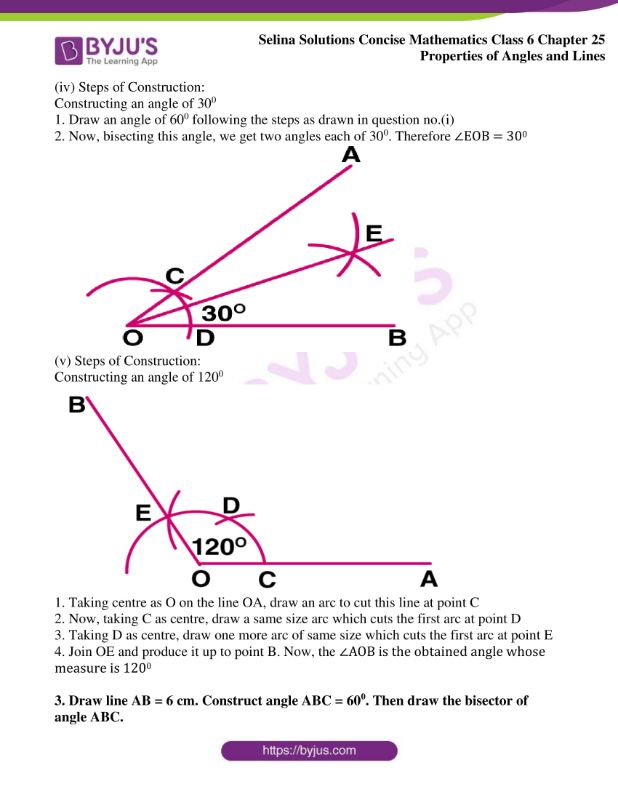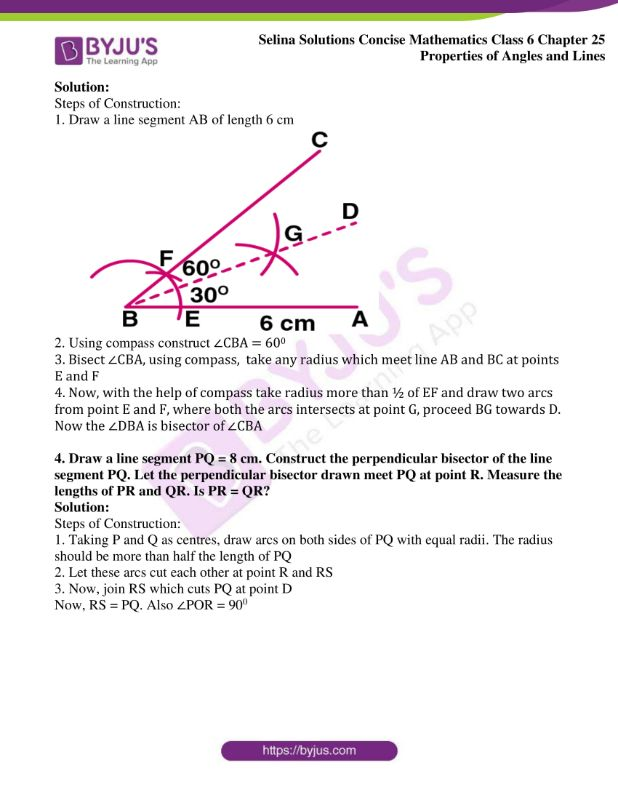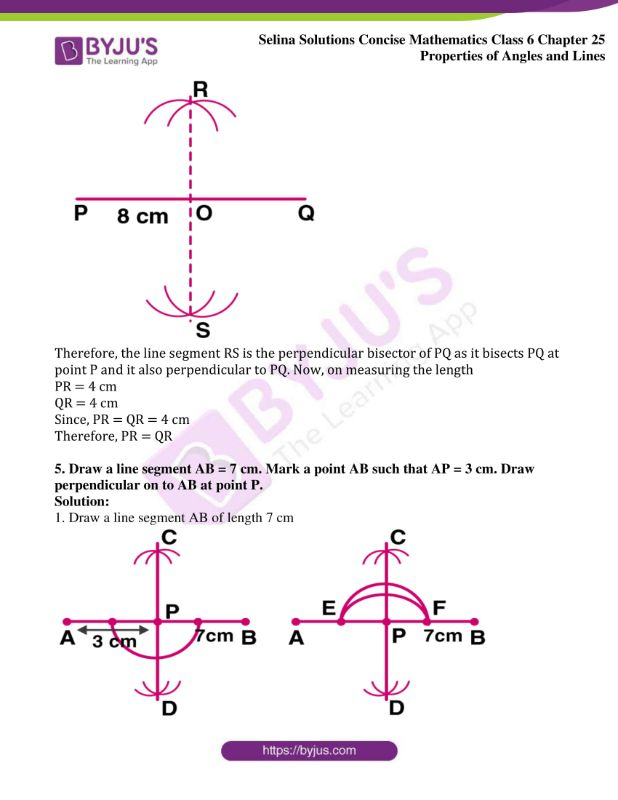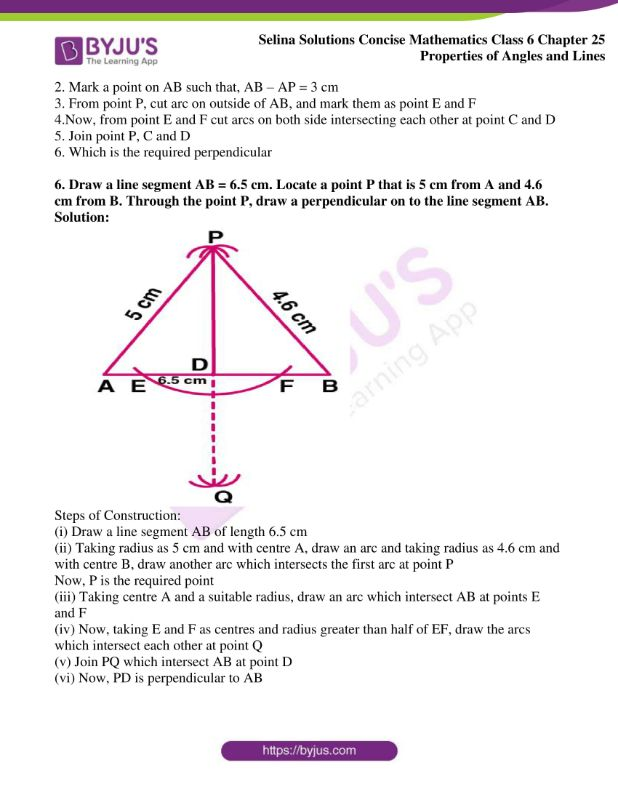### Access Selina Solutions Concise Mathematics Class 6 Chapter 25: Properties of Angles and Lines Exercise 25(C)

Exercise 25(C)

1. In your note-book copy the following angles using ruler and a pair compass only.

(i)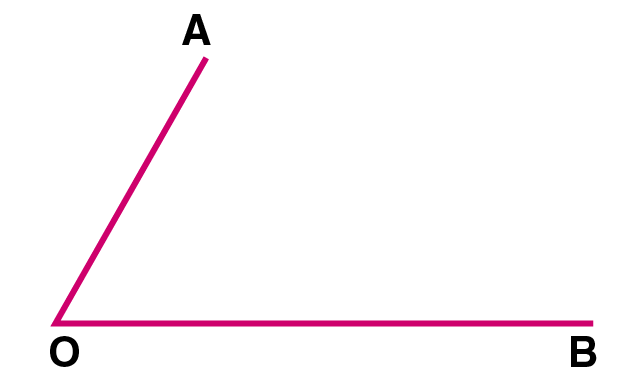(ii)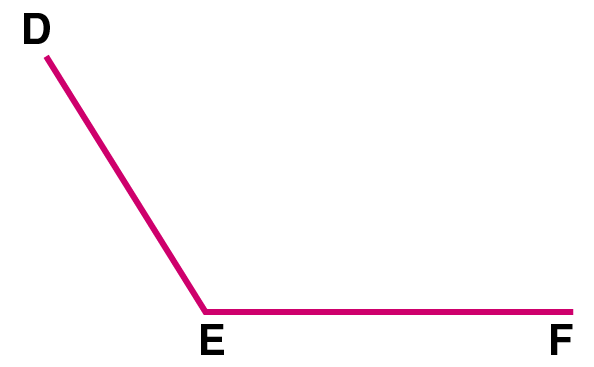(iii)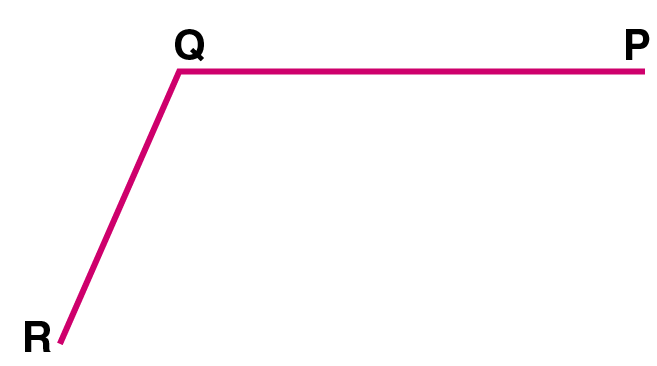Solution:

(i) Steps of Construction:

1. Draw line QR = OB at point Q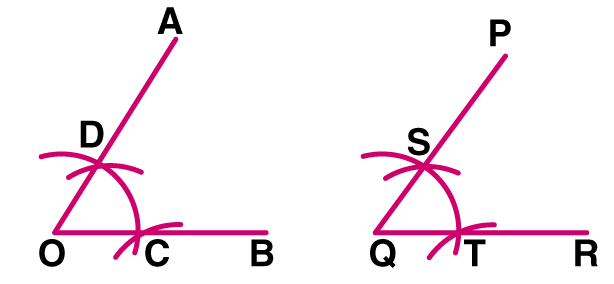2. Keeping O as centre, draw an arc of any suitable radius, to cut the arms of the angle at points C and D

3. Keeping Q as centre, draw the arc of the same size as drawn for C and D. Let this arc line cuts line QR at point T

4. With the help of compasses, take the distance equal to distance between C and D; and then taking T as centre, draw an arc which cuts the earlier arc at point S.

5. Join QS and produce up to a suitable point P. Now, the obtained ∠PQR , is the angle equal to the given ∠AOB

(ii) Steps of Construction:

1. Draw a line EF, at a point E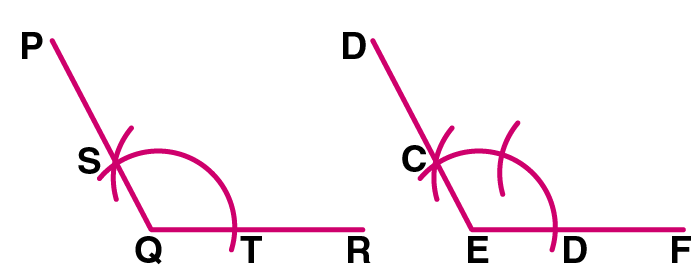2. Taking E as centre, draw an arc of any suitable radius, to cut the arms of the angle at points C and D

3. Taking Q as centre, draw an arc of the same size as drawn for points C and D. Let this arc cuts line QR at point T

4. With the help of compasses, take the distance equal to the distance between C and D; and then taking T as centre, draw an arc which cuts the earlier arc at point S

5. Join QS and produce up to a suitable point S. Now, the obtained ∠PQR is the angle equal to the the given ∠DEF

(iii) Steps of Construction:

1. Draw a line AB = QP at a point A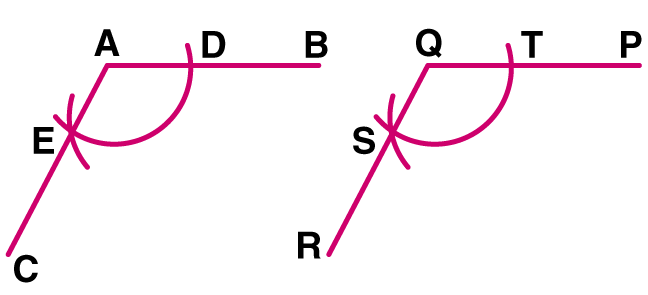2. Taking Q as centre, draw an arc of any suitable radius, to cut the arms of the angle T and S

3. Taking A as centre, draw an arc of the same size as drawn for points T and S. Let this arc cuts the line AB at point D

4. With the help of compasses, take the distance equal to the distance between T and S; and then taking D as centre, draw an arc which cuts the earlier arc at point E

5. Join AE produced up to a suitable point C. The obtained ∠BAC is the angle equal to the given ∠PQR

2. Construct the following angles, using ruler and a pair of compass only

(i) 600

(ii) 900

(iii) 450

(iv) 300

(v) 1200

Solution:

(i) Steps of Construction:

Constructing the angle of 600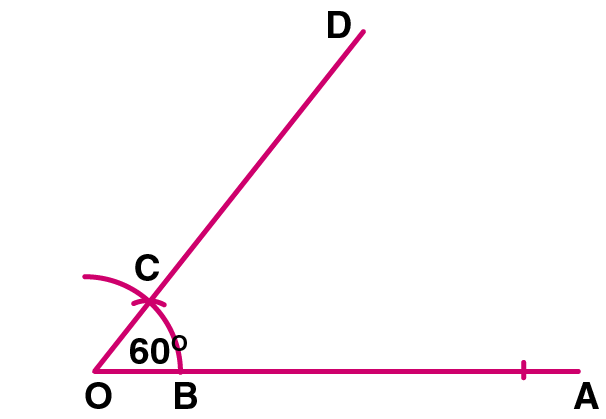1. Draw a line OA of any suitable length

2. Taking O as centre, draw an arc of any size to cut OA at point B

3. Now, taking B as centre, draw the same size arc, to cut the previous arc at point C

4. Join OC and produce up to a suitable point D. Then, the obtained ∠DOA is the angle of 600

(ii) Steps of Construction:

Constructing angle of 900

Let OA be the line and at point O, the angle of 900 is to be drawn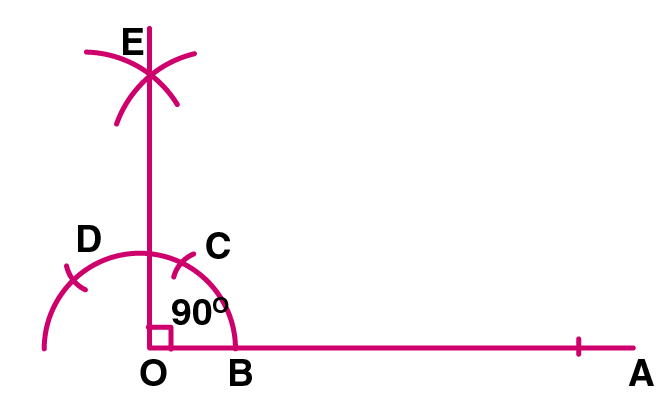1. Taking O as centre, draw an arc to cut the arm OA at point B

2. Taking B as centre, draw the same size arc to cut the previous arc at point C

3. Again with C as centre and with the same radius, draw one more arc to cut the previous arc at point D

4. Now, taking C and D as centres, draw two arcs of equal radii to cut each other at point E.

5. Join O and E. Then ∠AOE = 900 is obtained

(iii) Draw an angle of 900, following the steps as in question (ii) and bisect it. Each angle so obtained will be 450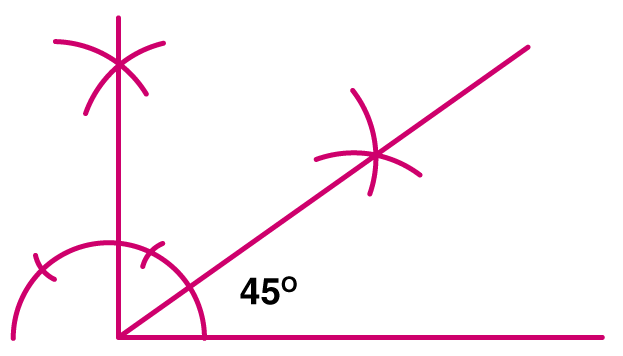(iv) Steps of Construction:

Constructing an angle of 300

1. Draw an angle of 600 following the steps as drawn in question no.(i)

2. Now, bisecting this angle, we get two angles each of 300. Therefore ∠EOB = 300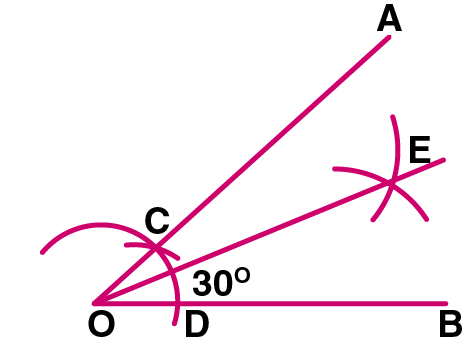(v) Steps of Construction:

Constructing an angle of 1200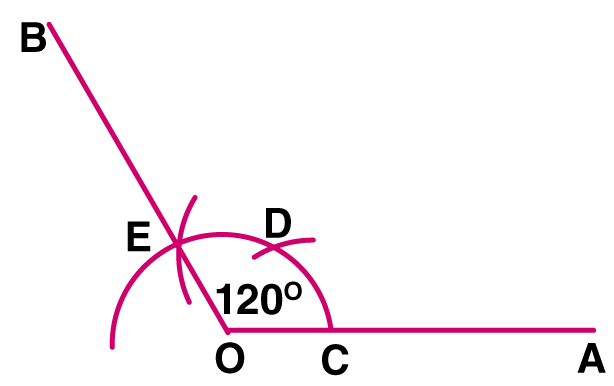1. Taking centre as O on the line OA, draw an arc to cut this line at point C

2. Now, taking C as centre, draw a same size arc which cuts the first arc at point D

3. Taking D as centre, draw one more arc of same size which cuts the first arc at point E

4. Join OE and produce it up to point B. Now, the ∠AOB is the obtained angle whose measure is 1200

3. Draw line AB = 6 cm. Construct angle ABC = 600. Then draw the bisector of angle ABC.

Solution:

Steps of Construction:

1. Draw a line segment AB of length 6 cm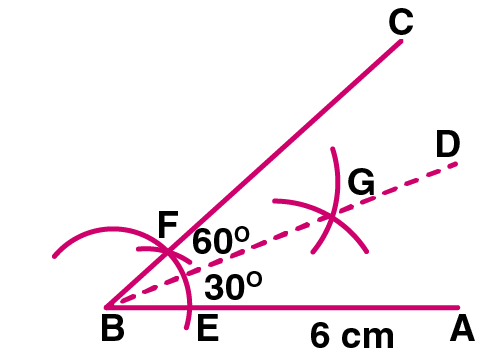2. Using compass construct ∠CBA = 600

3. Bisect ∠CBA, using compass, take any radius which meet line AB and BC at points E and F

4. Now, with the help of compass take radius more than ½ of EF and draw two arcs from point E and F, where both the arcs intersects at point G, proceed BG towards D. Now the ∠DBA is bisector of ∠CBA

4. Draw a line segment PQ = 8 cm. Construct the perpendicular bisector of the line segment PQ. Let the perpendicular bisector drawn meet PQ at point R. Measure the lengths of PR and QR. Is PR = QR?

Solution:

Steps of Construction:

1. Taking P and Q as centres, draw arcs on both sides of PQ with equal radii. The radius should be more than half the length of PQ

2. Let these arcs cut each other at point R and RS

3. Now, join RS which cuts PQ at point D

Now, RS = PQ. Also ∠POR = 900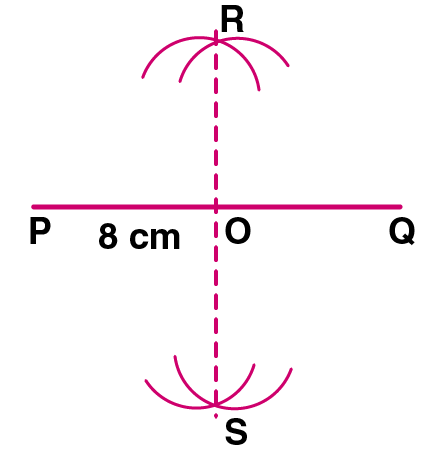Therefore, the line segment RS is the perpendicular bisector of PQ as it bisects PQ at point P and it also perpendicular to PQ. Now, on measuring the length

PR = 4 cm

QR = 4 cm

Since, PR = QR = 4 cm

Therefore, PR = QR

5. Draw a line segment AB = 7 cm. Mark a point AB such that AP = 3 cm. Draw perpendicular on to AB at point P.

Solution:

1. Draw a line segment AB of length 7 cm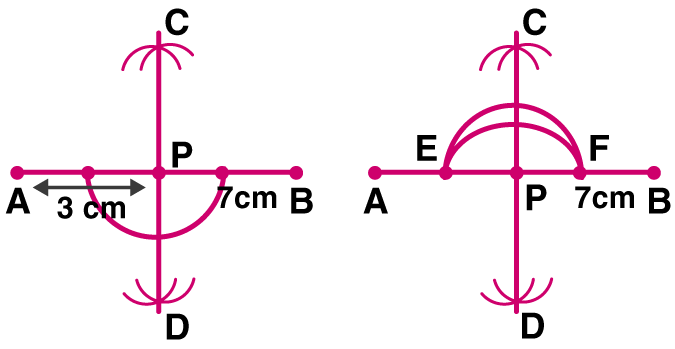2. Mark a point on AB such that, AB – AP = 3 cm

3. From point P, cut arc on outside of AB, and mark them as point E and F

4.Now, from point E and F cut arcs on both side intersecting each other at point C and D

5. Join point P, C and D

6. Which is the required perpendicular

6. Draw a line segment AB = 6.5 cm. Locate a point P that is 5 cm from A and 4.6 cm from B. Through the point P, draw a perpendicular on to the line segment AB.

Solution: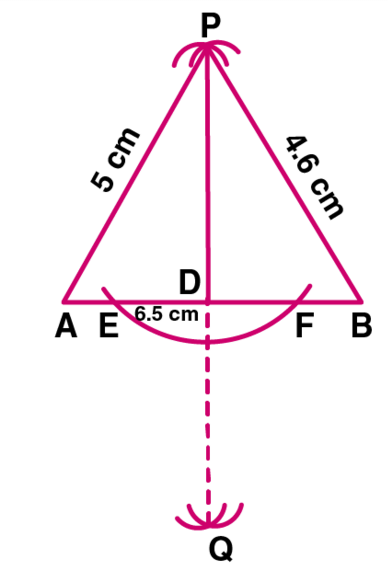Steps of Construction:

(i) Draw a line segment AB of length 6.5 cm

(ii) Taking radius as 5 cm and with centre A, draw an arc and taking radius as 4.6 cm and with centre B, draw another arc which intersects the first arc at point P

Now, P is the required point

(iii) Taking centre A and a suitable radius, draw an arc which intersect AB at points E and F

(iv) Now, taking E and F as centres and radius greater than half of EF, draw the arcs which intersect each other at point Q

(v) Join PQ which intersect AB at point D

(vi) Now, PD is perpendicular to AB